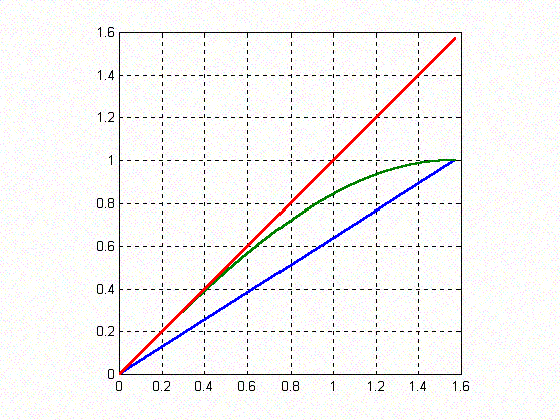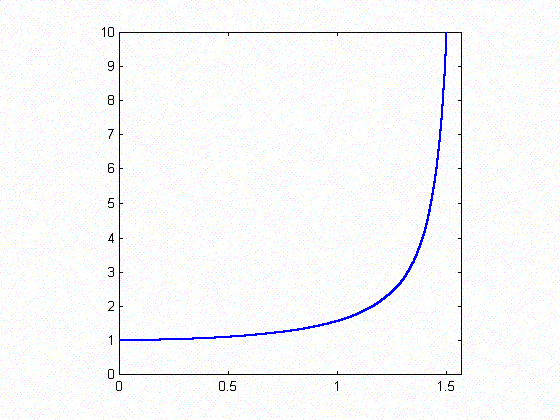#### You may also like### Shades of Fermat's Last Theorem

The familiar Pythagorean 3-4-5 triple gives one solution to (x-1)^n + x^n = (x+1)^n so what about other solutions for x an integer and n= 2, 3, 4 or 5?### Exhaustion

Find the positive integer solutions of the equation (1+1/a)(1+1/b)(1+1/c) = 2### Code to Zero

Find all 3 digit numbers such that by adding the first digit, the square of the second and the cube of the third you get the original number, for example 1 + 3^2 + 5^3 = 135.

# Climbing

##### Age 16 to 18Challenge Level

Thank you Andrei Lazanu from Tudor Vianu National College, Bucharest, Romania for this solution.

To solve the problem, I plotted three functions for $0 \leq x \leq \pi/2$. Below are the graphs of the three functions, all three plotted on the same figure with $y_1={2x\over \pi}$ in blue, $y_2 = \sin x$ in green and $y_3=x$ in red.As seen from the figure, the green graph (i.e. $y = \sin x$) is situated between the other two graphs in the range of $0 \leq x \leq \pi/2$. For all $x$ between 0 and $\pi/2$ the point $(x, \sin x)$ is above the point $(x, 2x/\pi)$ and hence $${2x\over \pi} \leq \sin x.$$ In this interval the graph of $\sin x$ lies below the line $y=x$ because this line meets the graph of $\sin x$ at the origin and the gradient of $\sin x$ is less than 1 for all $x$. Hence $\sin x \leq x$.

For the second part of the problem, I have to prove that: $${\tan a \over \tan b} < {a \over b}.$$ For any $a$ and $b$ in this interval, both $a$, $b$, $\tan a$ and $\tan b$ are positive, so that the original inequality is equivalent to the following one: $${\tan a \over a} < {\tan b \over b}$$ In the figure below the graph of function $y=\tan x/x$ is plotted for $0 < x < \pi/2$.I observe that the function is monotonically increasing. This means that for any $a < b$ (but remaining in the interval $0 < a < b < \pi/2$), $y(a) < y(b)$ as required.

Alternatively, from the graph of $y = \tan x$ in this interval, if $a < b$ then the gradient of line joining the origin the point $(a, \tan a)$ is less than the gradient of the line joining the origin to the point $(b, \tan b)$ so $${\tan a \over a} < {\tan b \over b}$$ and hence $${\tan a \over \tan b} < {a \over b}.$$